# Calculation of Analysis of Means limits

## 2007-03-06

This page shows some of the details for calculating an analysis of means (ANOM) chart.

### Resistivity example

This data set comes from the National Institutes of Standards and Technology.

The first three digits of the data values are constant, so you need to be careful in calculating means and standard deviations. Do not round with this data set. Resistivity measurements were recorded five times on five separate instruments. There is some concern that the instruments may have small but important differences in resistivity.

Instrument
1        2        3        4        5
196.3052 196.3042 196.1303 196.2795 196.2119
196.1240 196.3825 196.2005 196.1748 196.1051
196.1890 196.1669 196.2889 196.1494 196.1850
196.2569 196.3257 196.0343 196.1485 196.0052
196.3403 196.0422 196.1811 195.9885 196.2090


Compare these five instruments using an ANOM chart.

      mean         var      stdev
1 196.2431 0.007651577 0.08747329
2 196.2443 0.019037095 0.13797498
3 196.1670 0.008784212 0.09372413
4 196.1481 0.010863213 0.10422674
5 196.1432 0.007823043 0.08844797


The average of the five means is 196.1892 and the average of the five variances is 0.01083183. The square root of this value, 0.1040761, is the pooled standard deviation.

The formulas for the decision limits in an ANOM chart arewhere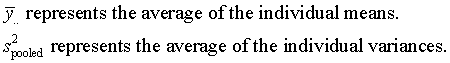In this example, I is 5 and N-I is 20. The value of h from the table of critical values for a balanced ANOM is 2.80. The ANOM decision limits areand the ANOM chart looks like this:All five means are inside the decision limits, so you would conclude that no individual mean differs from the overall average.

You can also use an ANOM chart for the standard deviations. I will not show the formulas or the calculations for this chart, but here is what it looks like:All standard deviations are inside the decision limits, so you would conclude that no individual standard deviation differs from the pooled standard deviation.

### Toy slingshot

I asked a group of three volunteers to collect some data on their accuracy at hitting a target with a toy slingshot. They took three shots with their dominant hand and measured the distance of each shot from the target. Then they took three shots with their non-dominant hand and took three shots at the target. Their results are recorded below

Name Shot1 Shot2 Shot3
J-D       62       20       14
J-N       21        9       37
A-D       20       24       37
A-N       43       40       26
M-D       17       75       29
M-N       27       21       59


My intention was to treat these six rows as if they represented six separate individuals (I did not have enough volunteers!). This is perhaps a bit of an oversimplification, and some time in the future I want to analyze the data as a two factor study. For this weblog entry, though, I want to plot these results using an analysis of means chart with a factor having six levels.

The summary statistics are easily computed.

Name   Mean      Var     Stdev
J-D   32.00   684.00     26.15
J-N   22.33   197.33     14.05
A-D   27.00    79.00      8.89
A-N   36.33    82.33      9.07
M-D   40.33   937.33     30.62
M-N   35.67   417.33     20.43


The average of the six means is 32.28. The average variance is 399.6, and the square root of this value, 19.99, represents the pooled standard deviation.

In this example, I is 6 and N is 18. The critical value h is 3.07. The upper decision limit is 64.62, as shown below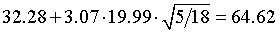and the lower decision limit is -0.06, as shown belowWe round the lower decision limit up to zero because a negative result is impossible in this experiment. Here is a graph showing the individual means and the ANOM limits.Even though there is some disparity in the mean values, these disparities are well within the limits of sampling error.

Although an ANOM chart for the standard deviations is theoretically possible, you should not calculate such a chart unless you have more observations contributing to each individual standard deviation.

### Hypothetical change in sample size

For a process with as much randomness as this, it may make sense to ask each individual to shoot at the target five or ten times. What would the decision limits look like if these individual means and standard deviations were based on ten runs rather than three?

In this case, the value of I would remain the same (6) but the value of N would increase to 60. The critical value for h would be 2.71. The upper decision limit is 47.92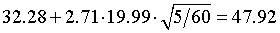and the lower decision limit is### Improvement in toy slingshot experiment

The group then worked on the process and made some improvements. Here is the data after the process was improved

J-D 52 12 18
J-N  5  2 34
A-D  9 22 17
A-N 22 19  8
M-D 25 29 10
M-N 27  3 15


The summary statistics for each group are

Name   Mean      Var     Stdev
J-D   27.33   465.33     21.57
J-N   13.67   312.33     17.67
A-D   16.00    43.00      6.56
A-N   16.33    54.33      7.37
M-D   21.33   100.33     10.02
M-N   15.00   144.00     12.00
Avg   18.28   186.56


The square root of the average variance, 13.65, represents the pooled standard deviation. The decision limits areand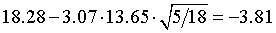which we round to zero. The ANOM chart is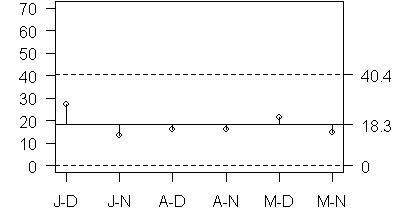Again, all data points are inside the decision limits.

### Analysis of proportions

The analysis simplifies somewhat if your data is a set of proportions rather than a set of means. You no longer need to compute a pooled standard deviation, but instead use a formula for variation that is a simple function of the average proportion. You also do not need to calculate degrees of freedom, and can treat them as an infinite number of degrees of freedom. This is effectively the same thing as replacing a t-distribution with a normal distribution. Here is the formula.A worker is asked to compare four different hearing tests to assure that they are of comparable difficulty.

Test-1 86%
Test-2 56%
Test-3 90%
Test-4 86%


The results represent the percentage of words out of fifty that are identified correctly. The average of these four proportions is 0.795. The decision limits are computed asand the ANOM chart looks like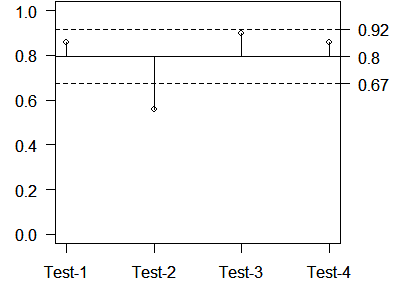The second test appears to be more difficult than average.

1. A different group of volunteers was asked to shoot a toy rocket at a target (data shown below). Six different people recorded their accuracy on two consecutive shots. Calculate an ANOM chart for this data.
A 14 39
B 60 20
C 26  9
D  9 12
E 36 21
F 53 18

1. The following data is fictional. Twenty separate laboratories were sent identical images of a sperm smear with exactly 100 sperm cells and were asked to estimate the proportion of normal cells on the image using WHO-3 standards (data shown below). Calculate an ANOM chart for these proportions. Don’t peek but the answers are available on a separate web page.
25 23 22 18 24 30 22 28 29 15
19 35 33 35 33 17 19 19 40 26


You can find an earlier version of this page on my old website.